## How to countif with multiple criteria in Excel?

In Excel, COUNTIF function may help us to calculate the number of a certain value in a list. But sometimes, we need to use multiple criteria for counting, this will be more complex, today, I will talk about some items for counting with multiple criteria.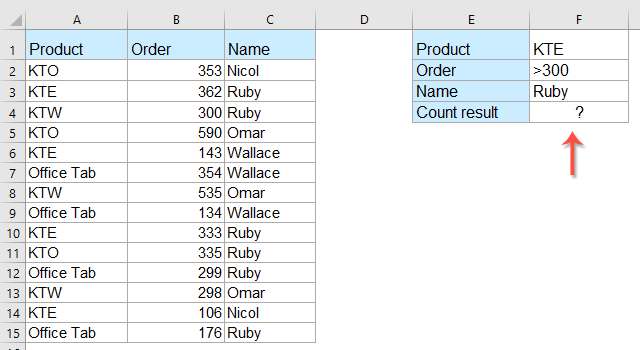#### Count cells with multiple criteria based on text value

For example, I have the following data which contains some products, and now I need to count the number of KTE and KTO which are populated in the same column, see screenshot: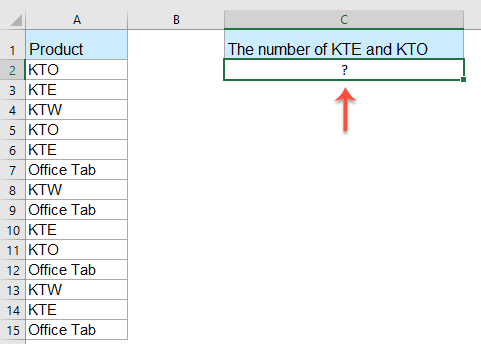To get the number of KTE and KTO, please enter this formula:

=COUNTIF(\$A\$2:\$A\$15,"KTE")+COUNTIF(\$A\$2:\$A\$15,"KTO")

And then press Enter key to get the number of these two products. See screenshot: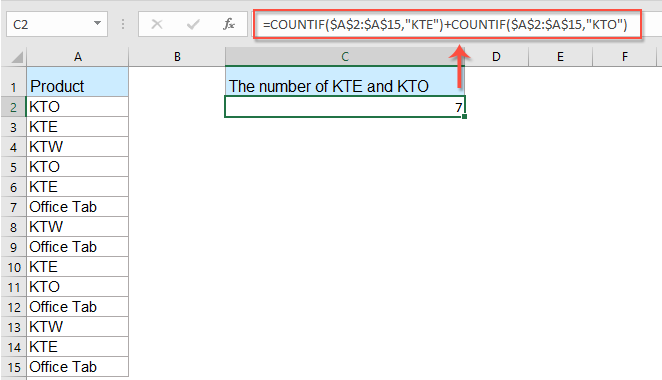Notes:

1. In the above formula: A2:A15 is the data range that you want to use, KTE and KTO are the criteria that you want to count.

2. If there are more than two criteria that you want to count in one column, just use =COUNTIF(range1, criteria1) + COUNTIF(range2, criteria2) + COUNTIF(range3, criteria3)+…

• Tips:
• Another compact formula also can help you to solve this problem: =SUMPRODUCT(COUNTIF(\$A\$2:\$A\$15,{"KTE";"KTO"})), and then press Enter key to get the result.
• And you can add the criteria just as =SUMPRODUCT(COUNTIF(range,{ "criteria1";"criteria2";"criteria3";"criteria4"…})).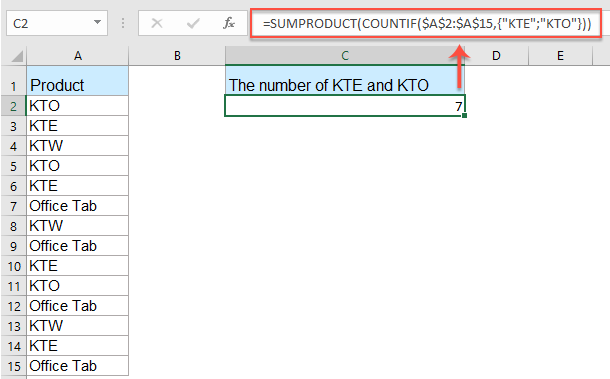#### Count cells with multiple criteria between two values

If you need to count the number of cells that the value is between two given numbers, how to solve this job in Excel?

Take the below screenshot as an example, I want to get the result of the number that is between 200 and 500. Please do with these formulas: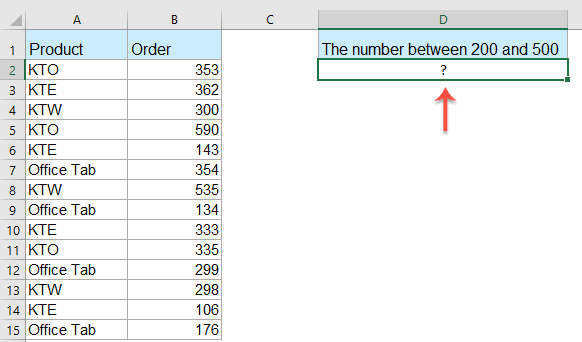Enter this formula into a blank cell where you want to locate the result:

=COUNTIF(\$B\$2:\$B\$15,">200")-COUNTIF(\$B\$2:\$B\$15,">500")

And then press Enter key to get the result as you need, see screenshot: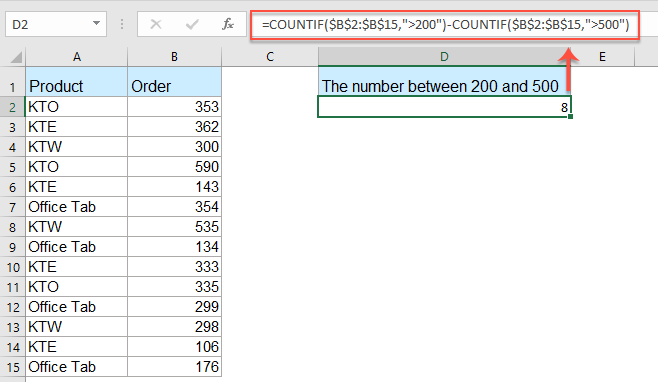Note: In the above formula:

• B2:B15 is the cell range that you want to use, >200 and >500 are the criteria you want to count the cells;
• the whole formula means, to find the number of cells that have a value greater than 200 and then subtract the count of cells with a value greater than 500.
• Tips:
• You can also apply the COUNTIFS function to deal with this task, please type this formula: =COUNTIFS(\$B\$2:\$B\$15,">200",\$B\$2:\$B\$15,"<500"), and then press Enter key to get the result.
• And you can add the criteria just as =COUNTIFS(range1,"criteria1",range2,"criteria2",range3,"criteria3",...).

#### Count cells with multiple criteria between two dates

To count the cells based on date range, the COUNTIF and COUNTIFS functions can do you a favor as well.

For example, I want to count the cell numbers that the date is between 5/1/2019 and 8/1/2019 in a column, please do as this:

Enter the below formula into a blank cell:

=COUNTIFS(\$B\$2:\$B\$15, ">=5/1/2019", \$B\$2:\$B\$15, "<=8/1/2019")

And then press Enter key to get the count number, see screenshot: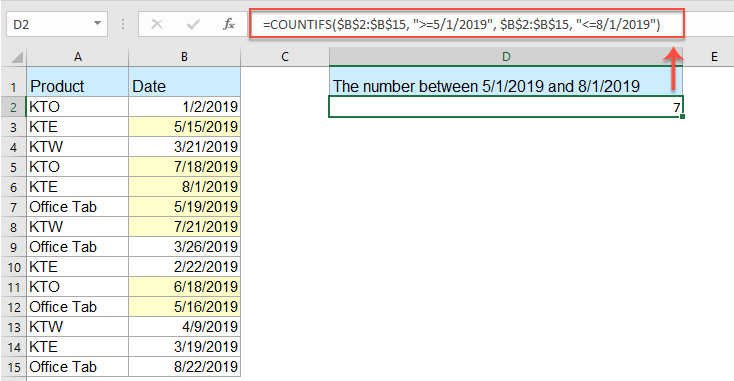Note: In the above formula:

• B2:B15 is the cell range that you want to use;
• >=5/1/2018 and <=8/1/2019 are the date criteria you want to count the cells;

Click to know more about the COUNTIF function...

#### Countif with multiple criteria in same column with a useful feature

If you have Kutools for Excel, with its Select Specific Cells feature, you can quickly select the cells with specific text or cells between two numbers or dates, and then get the number you need.

Tips:To apply this Select Specific Cells feature, firstly, you should download the Kutools for Excel, and then apply the feature quickly and easily.

After installing Kutools for Excel, please do as this:

1. Select the list of cells that you want to count cells based on criteria, and then click Kutools > Select > Select Specific Cells, see screenshot: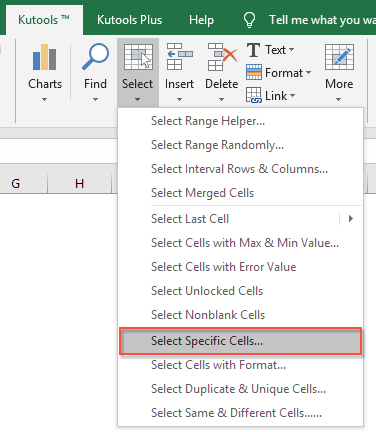2. In the Select Specific Cells dialog box, please set the operations as you need, and then click OK, the specific cells have been selected and the number of the cells are shown in the prompt box as below screenshots shown: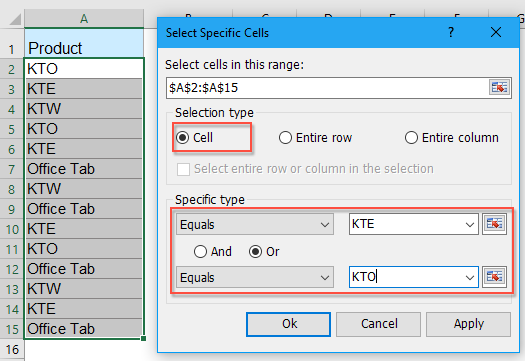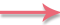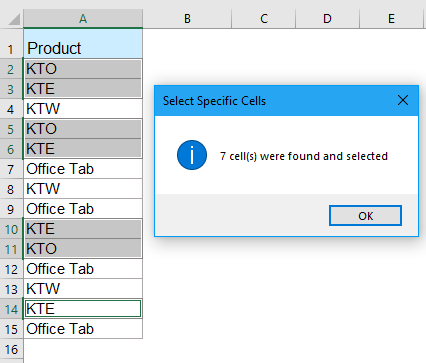Note: This feature also can help you to select and count the cells between two specific numbers or dates as the following screenshots shown: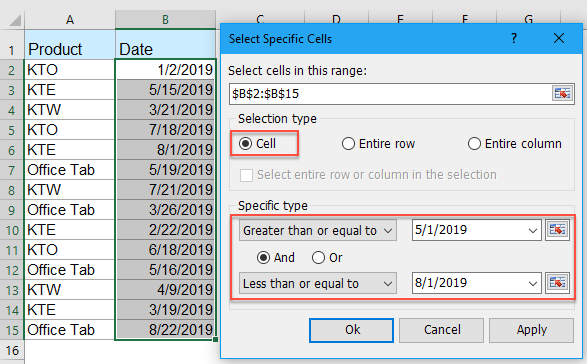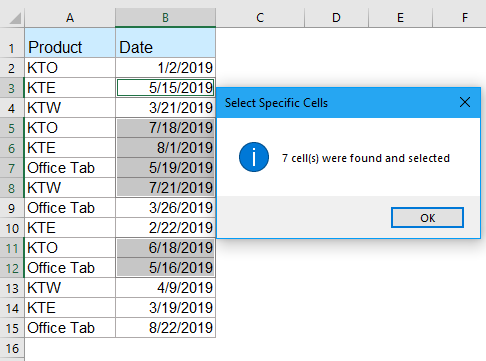#### Countif with multiple criteria in multiple columns

If there are multiple criteria in multiple columns, such as following screenshot shown, and I want to get the number of KTE whose order is greater than 300 and the name is Ruby.Please type this formula into the desired cell:

=COUNTIFS(\$A\$2:\$A\$15,"KTE",\$B\$2:\$B\$15,">300",\$C\$2:\$C\$15,"Ruby")

and then press Enter key to get the number of KTE which you need.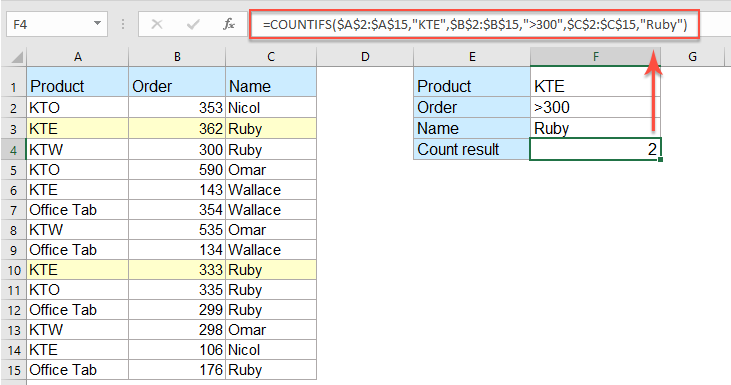Notes:

1. A2:A15 and KTE are the first range and criterion you need, B2:B15 and >300 are the second range and criterion you need, and the C2:C15 and Ruby are the third range and criterion you based on.

2. If there are more criteria you need based on, you just need to add the range and criteria within the formula, such as: =COUNTIFS(range1, criteria1, range2, criteria2, range3, criteria3, range4, criteria4, … )

• Tips:
• Here is another formula also can help you: =SUMPRODUCT(--(\$A\$2:\$A\$15="KTE"),--(\$B\$2:\$B\$15>300),--(\$C\$2:\$C\$15="Ruby")), and then press Enter key to get the result.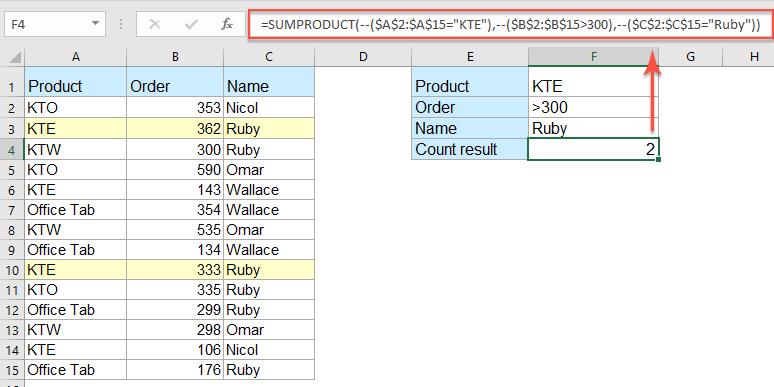Click to know more about the COUNTIFS function...

#### More relative count cells articles:

• Countif To Calculate The Percentage In Excel
• For example, I have a summary report of a research paper, and there are three options A, B, C, now I want to calculate the percentage of each these three options. That is to say, I need to know the option A accounts for the percentage of all options.
• Countif A Specific Value Across Multiple Worksheets
• Supposing, I have multiple worksheets which contain the following data, and now, I want to get the number of occurrence of a specific value “Excel” from theses worksheets. How could I count specific values across multiple worksheet?
• Countif Partial String/Substring Match In Excel
• It’s easy to countif cells filled with certain strings, but do you know how to countif cells containing only partial string or substrings in Excel? This article will introduce a couple of methods to solve it quickly.
• Count All Cells Except A Specific Value In Excel
• If you have the word "Apple" scattered among a list of values, now, you just want to count the number of cells which are not "Apple" to get the following result. In this article, I will introduce some methods to solve this task in Excel.
• Count Cells If One Of Multiple Criteria Met In Excel
• The COUNTIF function will help us count cells containing one criterion, and the COUNTIFS function can help up count cells containing a set of conditions or criteria in Excel. What if counting cells if contains one of multiple criteria? Here I will share the ways to count cells if contain X or Y or Z … etc. in Excel.

### Best Office Productivity Tools

 Popular Features: Find, Highlight or Identify Duplicates   |  Delete Blank Rows   |  Combine Columns or Cells without Losing Data   |   Round without Formula ... Super Lookup: Multiple Criteria VLookup  |   Multiple Value VLookup  |   VLookup Across Multiple Sheets   |   Fuzzy Lookup .... Advanced Drop-down List: Quickly Create Drop Down List   |  Dependent Drop Down List   |  Multi-select Drop Down List .... Column Manager: Add a Specific Number of Columns  |  Move Columns  |  Toggle Visibility Status of Hidden Columns  |  Compare Ranges & Columns ... Featured Features: Grid Focus   |  Design View   |   Big Formula Bar   |  Workbook & Sheet Manager   |  Resource Library (Auto Text)   |  Date Picker   |  Combine Worksheets   |  Encrypt/Decrypt Cells   |  Send Emails by List   |  Super Filter   |   Special Filter (filter bold/italic/strikethrough...) ... Top 15 Toolsets:  12 Text Tools (Add Text, Remove Characters, ...)   |   50+ Chart Types (Gantt Chart, ...)   |   40+ Practical Formulas (Calculate age based on birthday, ...)   |   19 Insertion Tools (Insert QR Code, Insert Picture from Path, ...)   |   12 Conversion Tools (Numbers to Words, Currency Conversion, ...)   |   7 Merge & Split Tools (Advanced Combine Rows, Split Cells, ...)   |   ... and more

Supercharge Your Excel Skills with Kutools for Excel, and Experience Efficiency Like Never Before. Kutools for Excel Offers Over 300 Advanced Features to Boost Productivity and Save Time.  Click Here to Get The Feature You Need The Most...#### Office Tab Brings Tabbed interface to Office, and Make Your Work Much Easier

• Enable tabbed editing and reading in Word, Excel, PowerPoint, Publisher, Access, Visio and Project.
• Open and create multiple documents in new tabs of the same window, rather than in new windows.
• Increases your productivity by 50%, and reduces hundreds of mouse clicks for you every day!No ratings yet. Be the first to rate!
This comment was minimized by the moderator on the site
I need to count the value has 2, The text is "A", to be counted has 2, excel formula
This comment was minimized by the moderator on the site
Muchas gracias <3
This comment was minimized by the moderator on the site
Thank you for this <3
This comment was minimized by the moderator on the site
hi,
what would the sum be if i needed to count a status of a person over a sheet?
example:
A B C D JAMES ABSENTJOHN PRESENTMIKE ABSENTJAMES PRESENT
Result required:
James absent = 1John absent = 0Mike absent =1
James present = 1John present = 1Mike present = 0

This comment was minimized by the moderator on the site
so basically count the uniquest in C that are not in B with a certain value in D
This comment was minimized by the moderator on the site
Hi,
I use this formulat =SUMPRODUCT((COUNTIF(B2:B1000,C2:C1000)=0)*(C2:C1000<>"")) to count uniques that dont appear in column bhow can i add another criteria in the formula that should count only a value in column D (new column)
This comment was minimized by the moderator on the site
Hi,
I need to count the different names in a single column which is repeated more than one time..
aaaaaaaaaaaaaaaabbbbbbbbbbbbbbbbbbbbbbbbcccccccccccccccccccc
i dont want to mention names in formula, because its many names in the column and i dont want pivot table also,I tried with this formula, but its counting the blank also..=SUMPRODUCT(1/COUNTIF(C10:C500,C10:C500&""))

Result what i need is -
aaaa - 4bbbb - 6cccc - 5

This comment was minimized by the moderator on the site
Hi, alwin,
To solve your problem, you should apply the following array formula:
=SUM(IF(\$A\$1:\$A\$11=C1,1,0)), after entering this formula, please press Ctrl + Shift + Enter keys together to get the correct result.
See the below screenshot:
This comment was minimized by the moderator on the site
Hi, I am wondering if someone can help me figure our how to combine COUNTIFS formulas. For example, I need to present this more efficiently:

=COUNTIFS(VAR1,”Yes”,VAR2,"2016",VAR3,"Q1",VAR4,PH,VAR5,"Pres") +
COUNTIFS(VAR1,”Yes”,VAR2,"2016",VAR3,"Q1",VAR4,"Both", VAR5,"Pres") +
COUNTIFS(VAR1,”Yes”,VAR2,"2016",VAR3,"Q1", VAR6,PH, VAR5,"Pres") +
COUNTIFS(VAR1,”Yes”,VAR2,"2016",VAR3,"Q1", VAR6,"Both", VAR5,"Pres")

So I need counts if VAR1 =Yes; VAR2 = 2016; VAR3=Q1; VAR5=Pres; AND VAR4= PH or Both; AND VAR6=PH or Both. I think the way I have it does the job, but I'm sure there is a more concise way to write the formula as it may get very long as I add criteria to it.
This comment was minimized by the moderator on the site
Great content, very helpful! Thank you so much for sharing this information!
This comment was minimized by the moderator on the site
hi, need to countif from two file with range and selection
=IF((COUNTIFS([SCCD.xlsx]open!\$AV:\$AV,">6",\$AV:\$AV,"<10"),[SCCD.xlsx]open!\$T:\$T,C2,[SCCD.xlsx]open!\$V:\$V,"SLAHOLD")+COUNTIFS([KTPH.xlsx]open!\$H:\$H,"SLAHOLD",[KTPH.xlsx]open!\$J:\$J,">6",!\$J:\$J,"<10",[KTPH.xlsx]open!\$G:\$G,C2))=0,"",COUNTIFS([SCCD.xlsx]open!\$AV:\$AV,">6",\$AV:\$AV,"<10"),[SCCD.xlsx]open!\$T:\$T,C2,[SCCD.xlsx]open!\$V:\$V,"SLAHOLD")+COUNTIFS([KTPH.xlsx]open!\$H:\$H,"SLAHOLD",[KTPH.xlsx]open!\$J:\$J,">6",\$J:\$J,"<10",[KTPH.xlsx]open!\$G:\$G,C2))